The margins and prediction packages are a combined effort to port the functionality of Stata’s (closed source) `margins` command to (open source) R. These tools provide ways of obtaining common quantities of interest from regression-type models. margins provides “marginal effects” summaries of models and prediction provides unit-specific and sample average predictions from models. Marginal effects are partial derivatives of the regression equation with respect to each variable in the model for each unit in the data; average marginal effects are simply the mean of these unit-specific partial derivatives over some sample. In ordinary least squares regression with no interactions or higher-order term, the estimated slope coefficients are marginal effects. In other cases and for generalized linear models, the coefficients are not marginal effects at least not on the scale of the response variable. margins therefore provides ways of calculating the marginal effects of variables to make these models more interpretable.

The major functionality of Stata’s `margins` command - namely the estimation of marginal (or partial) effects - is provided here through a single function, `margins()`. This is an S3 generic method for calculating the marginal effects of covariates included in model objects (like those of classes “lm” and “glm”). Users interested in generating predicted (fitted) values, such as the “predictive margins” generated by Stata’s `margins` command, should consider using `prediction()` from the sibling project, prediction.

## Motivation

Stata’s `margins` command is very simple and intuitive to use:

``````. import delimited mtcars.csv
. quietly reg mpg c.cyl##c.hp wt
. margins, dydx(*)
------------------------------------------------------------------------------
|            Delta-method
|      dy/dx   Std. Err.      t    P>|t|     [95% Conf. Interval]
-------------+----------------------------------------------------------------
cyl |   .0381376   .5998897     0.06   0.950    -1.192735     1.26901
hp |  -.0463187    .014516    -3.19   0.004     -.076103   -.0165343
wt |  -3.119815    .661322    -4.72   0.000    -4.476736   -1.762894
------------------------------------------------------------------------------
. marginsplot``````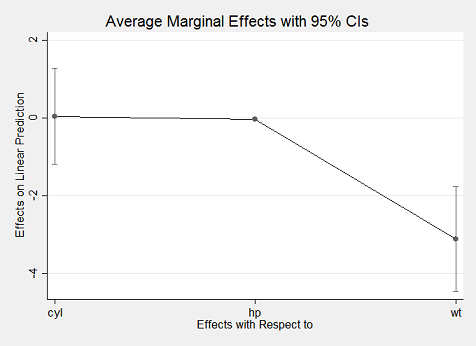With margins in R, replicating Stata’s results is incredibly simple using just the `margins()` method to obtain average marginal effects and its `summary()` method to obtain Stata-like output:

``````library("margins")
x <- lm(mpg ~ cyl * hp + wt, data = mtcars)
(m <- margins(x))``````
``## Average marginal effects``
``## lm(formula = mpg ~ cyl * hp + wt, data = mtcars)``
``````##      cyl       hp    wt
##  0.03814 -0.04632 -3.12``````
``summary(m)``
``````##  factor     AME     SE       z      p   lower   upper
##     cyl  0.0381 0.5999  0.0636 0.9493 -1.1376  1.2139
##      hp -0.0463 0.0145 -3.1909 0.0014 -0.0748 -0.0179
##      wt -3.1198 0.6613 -4.7176 0.0000 -4.4160 -1.8236``````

With the exception of differences in rounding, the above results match identically what Stata’s `margins` command produces. A slightly more concise expression relies on the syntactic sugar provided by `margins_summary()`:

``margins_summary(x)``
``````##  factor     AME     SE       z      p   lower   upper
##     cyl  0.0381 0.5999  0.0636 0.9493 -1.1376  1.2139
##      hp -0.0463 0.0145 -3.1909 0.0014 -0.0748 -0.0179
##      wt -3.1198 0.6613 -4.7176 0.0000 -4.4160 -1.8236``````

Using the `plot()` method also yields an aesthetically similar result to Stata’s `marginsplot`:

``plot(m)``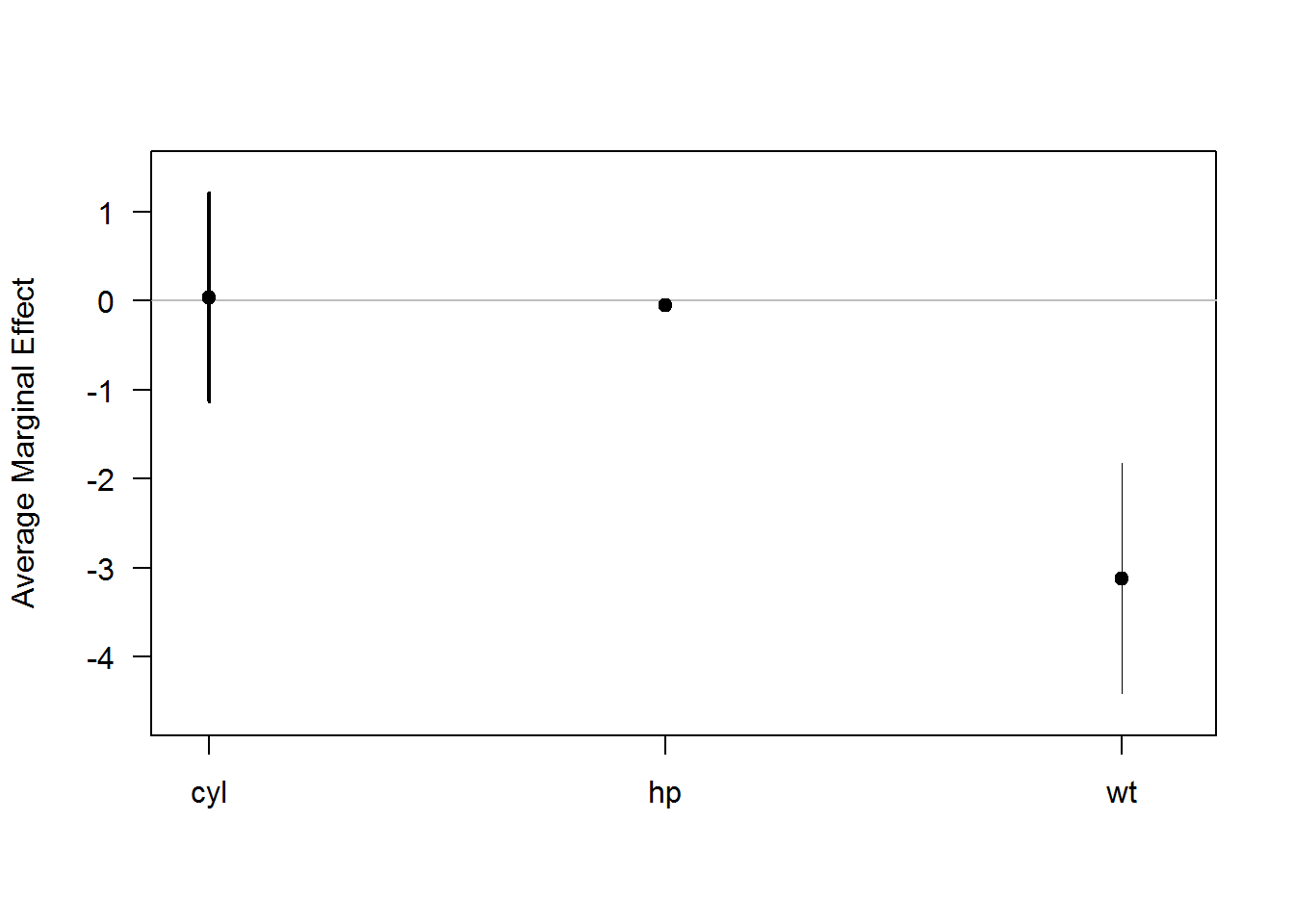## Using Optional Arguments in `margins()`

margins is intended as a port of (some of) the features of Stata’s `margins` command, which includes numerous options for calculating marginal effects at the mean values of a dataset (i.e., the marginal effects at the mean), an average of the marginal effects at each value of a dataset (i.e., the average marginal effect), marginal effects at representative values, and any of those operations on various subsets of a dataset. (The functionality of Stata’s command to produce predictive margins is not ported, as this is easily obtained from the prediction package.) In particular, Stata provides the following options:

• `at`: calculate marginal effects at (potentially representative) specified values (i.e., replacing observed values with specified replacement values before calculating marginal effects)
• `atmeans`: calculate marginal effects at the mean (MEMs) of a dataset rather than the default behavior of calculating average marginal effects (AMEs)
• `over`: calculate marginal effects (including MEMs and/or AMEs at observed or specified values) on subsets of the original data (e.g., the marginal effect of a treatment separately for men and women)

The `at` argument has been translated into `margins()` in a very similar manner. It can be used by specifying a list of variable names and specified values for those variables at which to calculate marginal effects, such as `margins(x, at = list(hp=150))`. When using `at`, `margins()` constructs modified datasets - using `build_datalist()` - containing the specified values and calculates marginal effects on each modified dataset, `rbind`-ing them back into a single “margins” object.

Stata’s `atmeans` argument is not implemented in `margins()` for various reasons, including because it is possible to achieve the effect manually through an operation like `data\$var <- mean(data\$var, na.rm = TRUE)` and passing the modified data frame to `margins(x, data = data)`.

At present, `margins()` does not implement the `over` option. The reason for this is also simple: R already makes data subsetting operations quite simple using simple `[` extraction. If, for example, one wanted to calculate marginal effects on subsets of a data frame, those subsets can be passed directly to `margins()` via the `data` argument (as in a call to `prediction()`).

The rest of this vignette shows how to use `at` and `data` to obtain various kinds of marginal effects, and how to use plotting functions to visualize those inferences.

### Average Marginal Effects and Average Partial Effects

``library("margins")``

We’ll use a simple example regression model based on the built-in `mtcars` dataset:

``x <- lm(mpg ~ cyl + hp * wt, data = mtcars)``

To obtain average marginal effects (AMEs), we simply call `margins()` on the model object created by `lm()`:

``margins(x)``
``## Average marginal effects``
``## lm(formula = mpg ~ cyl + hp * wt, data = mtcars)``
``````##      cyl       hp     wt
##  -0.3652 -0.02527 -3.838``````

The result is a data frame with special class `"margins"`. `"margins"` objects are printed in a tidy summary format, by default, as you can see above. The only difference between a `"margins"` object and a regular data frame are some additional data frame-level attributes that dictate how the object is printed.

The default method calculates marginal effects for all variables included in the model (ala Stata’s `, dydx(*)` option). To limit calculation to only a subset of variables, use the `variables` argument:

``summary(margins(x, variables = "hp"))``
``````##  factor     AME     SE       z      p   lower   upper
##      hp -0.0253 0.0105 -2.4046 0.0162 -0.0459 -0.0047``````

In an ordinary least squares regression, there is really only one way of examining marginal effects (that is, on the scale of the outcome variable). In a generalized linear model (e.g., logit), however, it is possible to examine true “marginal effects” (i.e., the marginal contribution of each variable on the scale of the linear predictor) or “partial effects” (i.e., the contribution of each variable on the outcome scale, conditional on the other variables involved in the link function transformation of the linear predictor). The latter are the default in `margins()`, which implicitly sets the argument `margins(x, type = "response")` and passes that through to `prediction()` methods. To obtain the former, simply set `margins(x, type = "link")`. There’s some debate about which of these is preferred and even what to call the two different quantities of interest. Regardless of all of that, here’s how you obtain either:

``x <- glm(am ~ cyl + hp * wt, data = mtcars, family = binomial)``
``## Warning: glm.fit: fitted probabilities numerically 0 or 1 occurred``
``margins(x, type = "response") # the default``
``## Average marginal effects``
``## glm(formula = am ~ cyl + hp * wt, family = binomial, data = mtcars)``
``````##      cyl       hp      wt
##  0.02156 0.002667 -0.5158``````
``margins(x, type = "link")``
``````## Average marginal effects
## glm(formula = am ~ cyl + hp * wt, family = binomial, data = mtcars)``````
``````##     cyl      hp     wt
##  0.5156 0.05151 -12.24``````

Note that some other packages available for R, as well as Stata’s `margins` and `mfx` packages enable calculation of so-called “marginal effects at means” (i.e., the marginal effect for a single observation that has covariate values equal to the means of the sample as a whole). The substantive interpretation of these is fairly ambiguous. While it was once common practice to estimate MEMs - rather than AMEs or MERs - this is now considered somewhat inappropriate because it focuses on cases that may not exist (e.g., the average of a 0/1 variable is not going to reflect a case that can actually exist in reality) and we are often interested in the effect of a variable at multiple possible values of covariates, rather than an arbitrarily selected case that is deemed “typical” in this way. As such, `margins()` defaults to reporting AMEs, unless modified by the `at` argument to calculate average “marginal effects for representative cases” (MERs). MEMs could be obtained by manually specifying `at` for every variable in a way that respects the variables classes and inherent meaning of the data, but that functionality is not demonstrated here.

### Using the `at` Argument

The `at` argument allows you to calculate marginal effects at representative cases (sometimes “MERs”) or marginal effects at means - or any other statistic - (sometimes “MEMs”), which are marginal effects for particularly interesting (sets of) observations in a dataset. This differs from marginal effects on subsets of the original data (see the next section for a demonstration of that) in that it operates on a modified set of the full dataset wherein particular variables have been replaced by specified values. This is helpful because it allows for calculation of marginal effects for counterfactual datasets (e.g., what if all women were instead men? what if all democracies were instead autocracies? what if all foreign cars were instead domestic?).

As an example, if we wanted to know if the marginal effect of horsepower (`hp`) on fuel economy differed across different types of automobile transmissions, we could simply use `at` to obtain separate marginal effect estimates for our data as if every car observation were a manual versus if every car observation were an automatic. The output of `margins()` is a simplified summary of the estimated marginal effects across the requested variable levels/combinations specified in `at`:

``````x <- lm(mpg ~ cyl + wt + hp * am, data = mtcars)
margins(x, at = list(am = 0:1))``````
``## Average marginal effects at specified values``
``## lm(formula = mpg ~ cyl + wt + hp * am, data = mtcars)``
``````##  at(am)     cyl     wt        hp    am
##       0 -0.9339 -2.812 -0.008945 1.034
##       1 -0.9339 -2.812 -0.026392 1.034``````

Because of the `hp * am` interaction in the regression, the marginal effect of horsepower differs between the two sets of results. We can also specify more than one variable to `at`, creating a potentially long list of marginal effects results. For example, we can produce marginal effects at both levels of `am` and the values from the five-number summary (minimum, Q1, median, Q3, and maximum) of observed values of `hp`. This produces 2 * 5 = 10 sets of marginal effects estimates:

``margins(x, at = list(am = 0:1, hp = fivenum(mtcars\$hp)))``
``## Average marginal effects at specified values``
``## lm(formula = mpg ~ cyl + wt + hp * am, data = mtcars)``
``````##  at(am) at(hp)     cyl     wt        hp      am
##       0     52 -0.9339 -2.812 -0.008945  2.6864
##       1     52 -0.9339 -2.812 -0.026392  2.6864
##       0     96 -0.9339 -2.812 -0.008945  1.9188
##       1     96 -0.9339 -2.812 -0.026392  1.9188
##       0    123 -0.9339 -2.812 -0.008945  1.4477
##       1    123 -0.9339 -2.812 -0.026392  1.4477
##       0    180 -0.9339 -2.812 -0.008945  0.4533
##       1    180 -0.9339 -2.812 -0.026392  0.4533
##       0    335 -0.9339 -2.812 -0.008945 -2.2509
##       1    335 -0.9339 -2.812 -0.026392 -2.2509``````

Because this is a linear model, the marginal effects of `cyl` and `wt` do not vary across levels of `am` or `hp`. The minimum and Q1 value of `hp` are also the same, so the marginal effects of `am` are the same in the first two results. As you can see, however, the marginal effect of `hp` differs when `am == 0` versus `am == 1` (first and second rows) and the marginal effect of `am` differs across levels of `hp` (e.g., between the first and third rows). As should be clear, the `at` argument is incredibly useful for getting a better grasp of the marginal effects of different covariates.

This becomes especially apparent when a model includes power-terms (or any other alternative functional form of a covariate). Consider, for example, the simple model of fuel economy as a function of weight, with weight included as both a first- and second-order term:

``````x <- lm(mpg ~ wt + I(wt^2), data = mtcars)
summary(x)``````
``````##
## Call:
## lm(formula = mpg ~ wt + I(wt^2), data = mtcars)
##
## Residuals:
##    Min     1Q Median     3Q    Max
## -3.483 -1.998 -0.773  1.462  6.238
##
## Coefficients:
##             Estimate Std. Error t value Pr(>|t|)
## (Intercept)  49.9308     4.2113  11.856 1.21e-12 ***
## wt          -13.3803     2.5140  -5.322 1.04e-05 ***
## I(wt^2)       1.1711     0.3594   3.258  0.00286 **
## ---
## Signif. codes:  0 '***' 0.001 '**' 0.01 '*' 0.05 '.' 0.1 ' ' 1
##
## Residual standard error: 2.651 on 29 degrees of freedom
## Multiple R-squared:  0.8191, Adjusted R-squared:  0.8066
## F-statistic: 65.64 on 2 and 29 DF,  p-value: 1.715e-11``````

Looking only at the regression results table, it is actually quite difficult to understand the effect of `wt` on fuel economy because it requires performing mental multiplication and addition on all possible values of `wt`. Using the `at` option to margins, you could quickly obtain a sense of the average marginal effect of `wt` at a range of plausible values:

``margins(x, at = list(wt = fivenum(mtcars\$wt)))``
``## Average marginal effects at specified values``
``## lm(formula = mpg ~ wt + I(wt^2), data = mtcars)``
``````##  at(wt)      wt
##   1.513 -9.8366
##   2.542 -7.4254
##   3.325 -5.5926
##   3.650 -4.8314
##   5.424 -0.6764``````

The marginal effects in the first column of results reveal that the average marginal effect of `wt` is large and negative except when `wt` is very large, in which case it has an effect not distinguishable from zero. We can easily plot these results using the `cplot()` function to see the effect visually in terms of either predicted fuel economy or the marginal effect of `wt`:

``cplot(x, "wt", what = "prediction", main = "Predicted Fuel Economy, Given Weight")``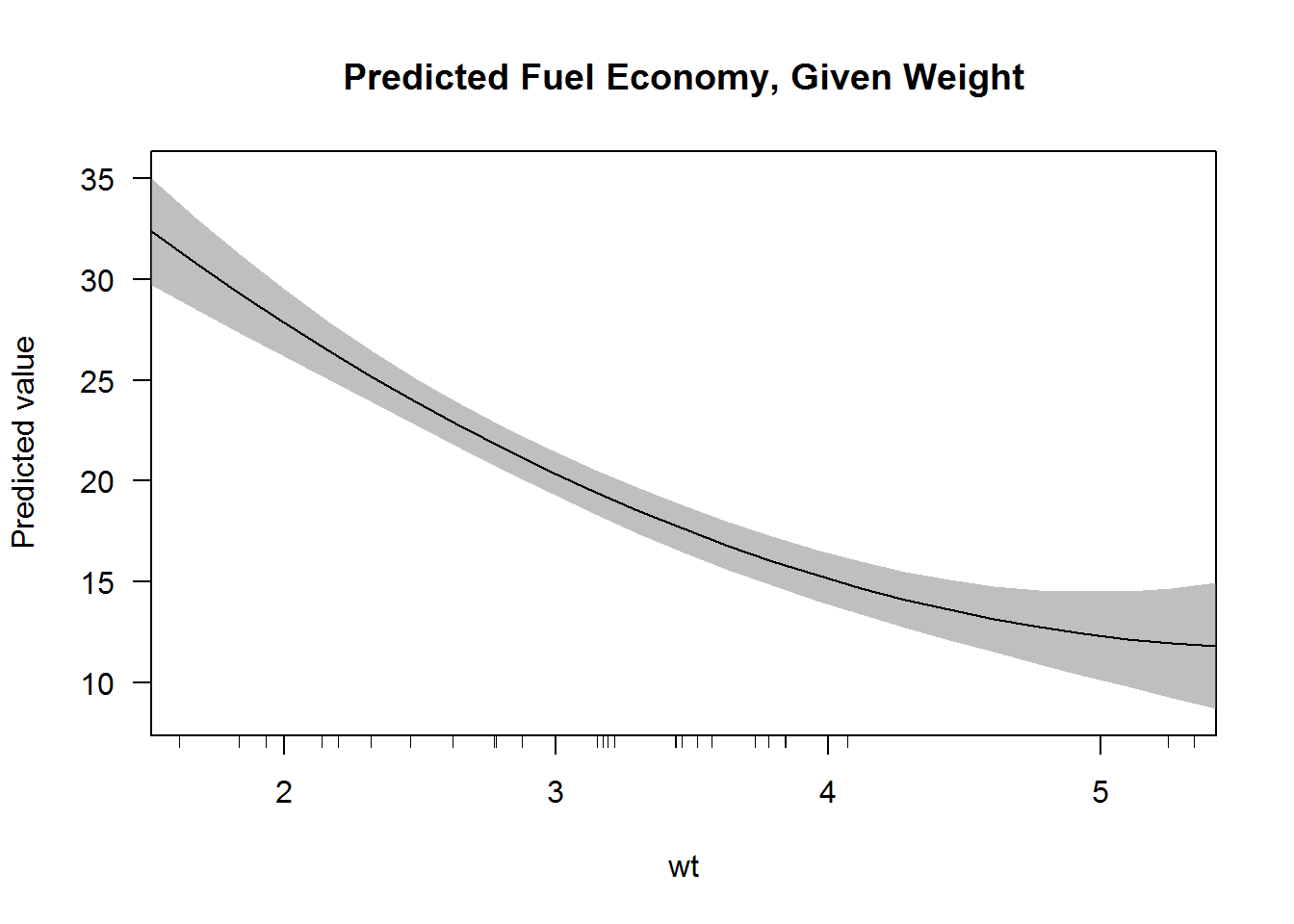``cplot(x, "wt", what = "effect", main = "Average Marginal Effect of Weight")``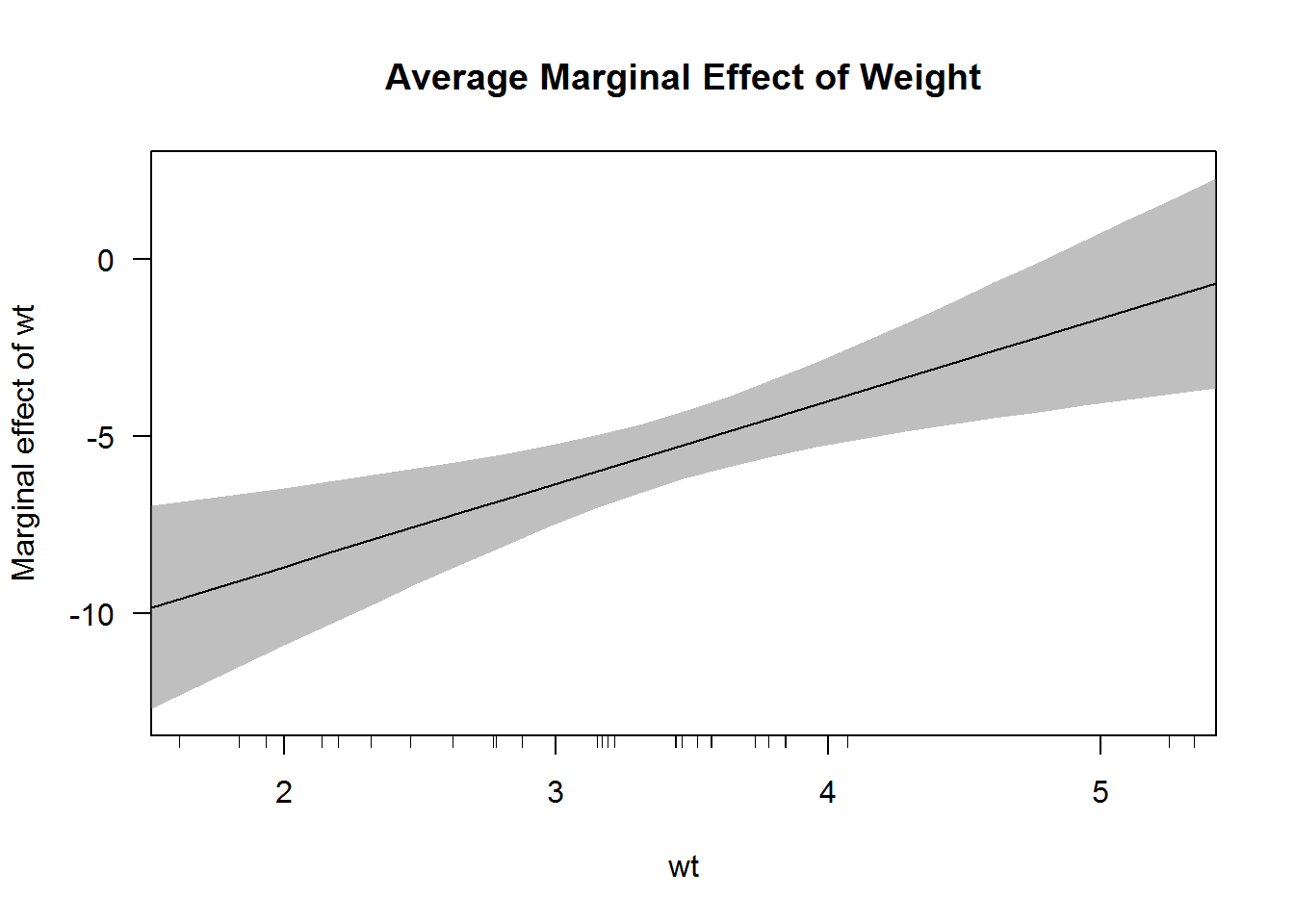A really nice feature of Stata’s margins command is that it handles factor variables gracefully. This functionality is difficult to emulate in R, but the `margins()` function does its best. Here we see the marginal effects of a simple regression that includes a factor variable:

``````x <- lm(mpg ~ factor(cyl) * hp + wt, data = mtcars)
margins(x)``````
``## Average marginal effects``
``## lm(formula = mpg ~ factor(cyl) * hp + wt, data = mtcars)``
``````##        hp    wt  cyl6   cyl8
##  -0.04475 -3.06 1.473 0.8909``````

`margins()` recognizes the factor and displays the marginal effect for each level of the factor separately. (Caveat: this may not work with certain `at` specifications, yet.)

### Subsetting

Stata’s `margins` command includes an `over()` option, which allows you to very easily calculate marginal effects on subsets of the data (e.g., separately for men and women). This is useful in Stata because the program only allows one dataset in memory. Because R does not impose this restriction and further makes subsetting expressions very simple, that feature is not really useful and can be achieved using standard subsetting notation in R:

``````x <- lm(mpg ~ factor(cyl) * am + hp + wt, data = mtcars)
# automatic vehicles
margins(x, data = mtcars[mtcars\$am == 0, ])``````
``## Average marginal effects``
``## lm(formula = mpg ~ factor(cyl) * am + hp + wt, data = mtcars)``
``````##     am       hp     wt   cyl6   cyl8
##  1.706 -0.03115 -2.441 -1.715 -1.586``````
``````# manual vehicles
margins(x, data = mtcars[mtcars\$am == 1, ])``````
``````## Average marginal effects
## lm(formula = mpg ~ factor(cyl) * am + hp + wt, data = mtcars)``````
``````##     am       hp     wt   cyl6   cyl8
##  2.167 -0.03115 -2.441 -4.218 -2.656``````

Because a `"margins"` object is just a data frame, it is also possible to obtain the same result by subsetting the output of `margins()`:

``````m <- margins(x)
split(m, m\$am)``````
``## \$`0```
``## Average marginal effects``
``## lm(formula = mpg ~ factor(cyl) * am + hp + wt, data = mtcars)``
``````##     am       hp     wt   cyl6   cyl8
##  1.706 -0.03115 -2.441 -1.715 -1.586
##
## \$`1```````
``````## Average marginal effects
## lm(formula = mpg ~ factor(cyl) * am + hp + wt, data = mtcars)``````
``````##     am       hp     wt   cyl6   cyl8
##  2.167 -0.03115 -2.441 -4.218 -2.656``````

## Marginal Effects Plots

Using `margins()` to calculate marginal effects enables several kinds of plotting. The built-in `plot()` method for objects of class `"margins"` creates simple diagnostic plots for examining the output of `margins()` in visual rather than tabular format. It is also possible to use the output of `margins()` to produce more typical marginal effects plots that show the marginal effect of one variable across levels of another variable. This section walks through the `plot()` method and then shows how to produce marginal effects plots using base graphics.

### The `plot()` method for “margins” objects

The margins package implements a `plot()` method for objects of class `"margins"` (seen above). This produces a plot similar (in spirit) to the output of Stata’s `marginsplot`. It is highly customizable, but is meant primarily as a diagnostic tool to examine the results of `margins()`. It simply produces, by default, a plot of marginal effects along with 95% confidence intervals for those effects. The confidence level can be modified using the `levels` argument, which is vectorized to allow multiple levels to be specified simultaneously.

There are two common ways of visually representing the substantive results of a regression model: (1) fitted values plots, which display the fitted conditional mean outcome across levels of a covariate, and (2) marginal effects plots, which display the estimated marginal effect of a variable across levels of a covariate. This section discusses both approaches.

Fitted value plots can be created using `cplot()` (to provide conditional predicted value plots or conditional effect plots) and both the `persp()` method and `image()` method for `"lm"` objects, which display the same type of relationships in three-dimensions (i.e., across two conditioning covariates).

For example, we can use `cplot()` to quickly display the predicted fuel economy of a vehicle from a model:

``````x <- lm(mpg ~ cyl + wt * am, data = mtcars)
cplot(x, "cyl")``````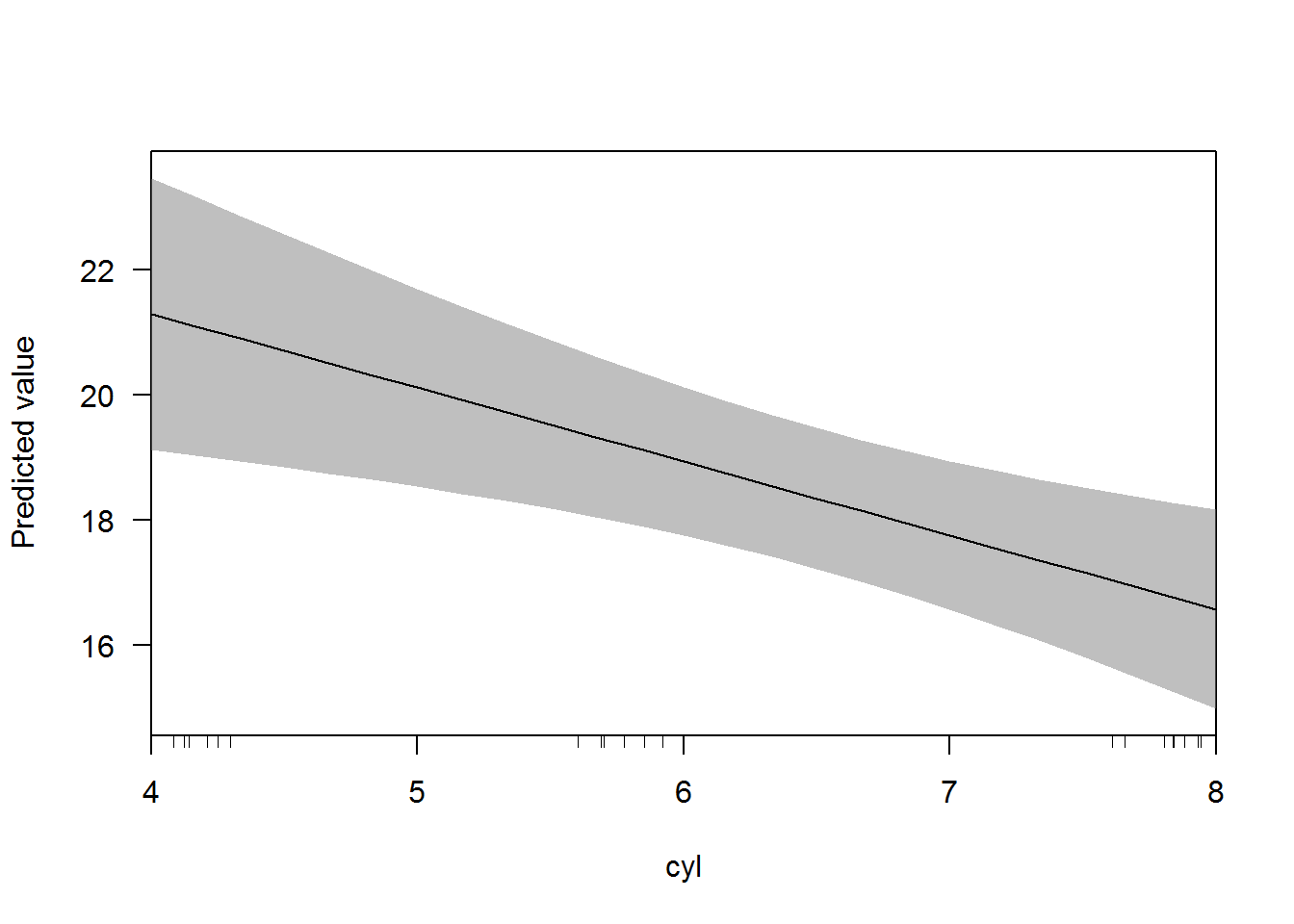``cplot(x, "wt")``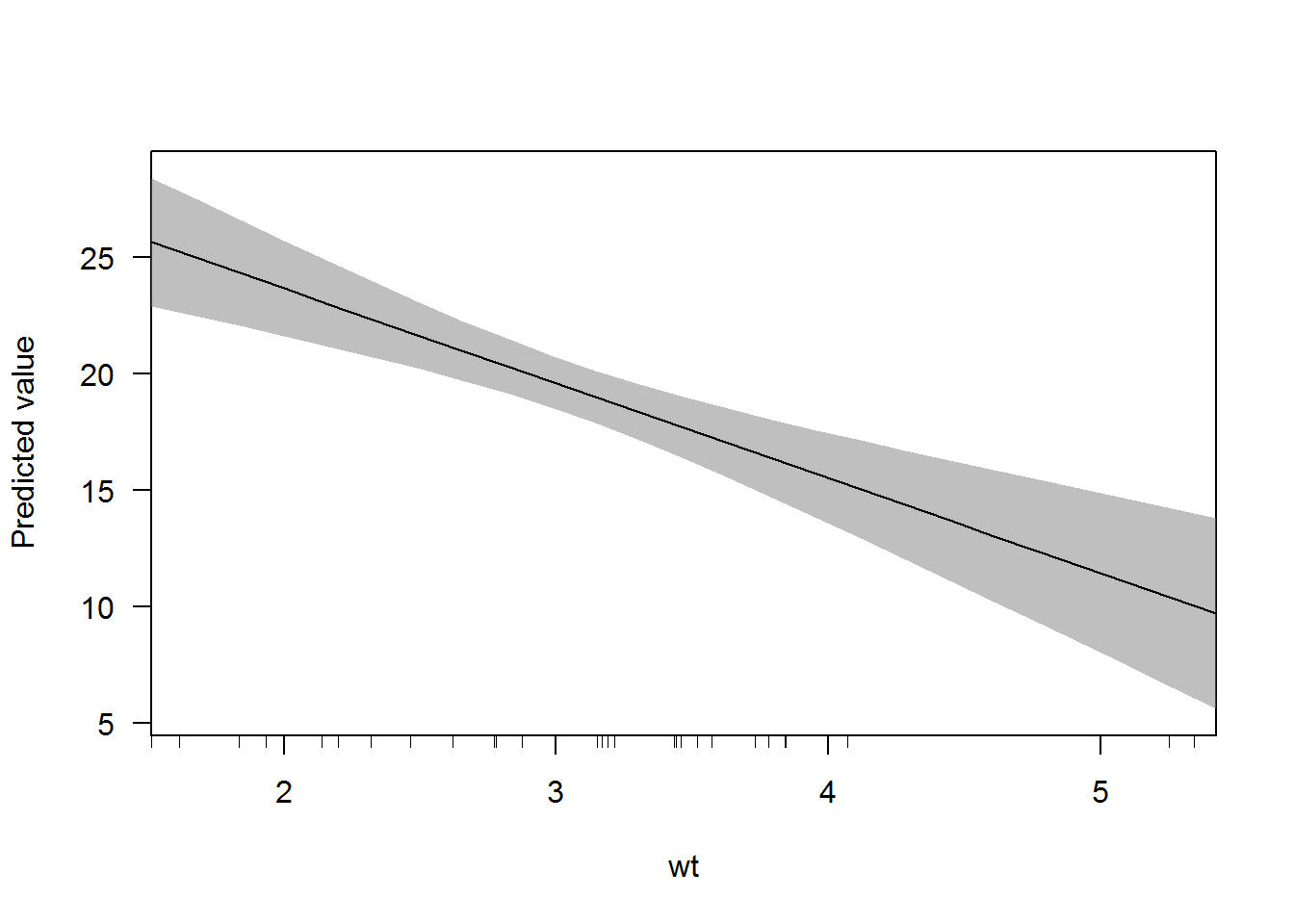The slopes of the predicted value lines are the marginal effect of `wt` when `am == 0` and `am == 1`. We can obtain these slopes using `margins()` and specifying the `at` argument:

``margins(x, at = list(am = 0:1))``
``## Average marginal effects at specified values``
``## lm(formula = mpg ~ cyl + wt * am, data = mtcars)``
``````##  at(am)    cyl     wt     am
##       0 -1.181 -2.369 -1.566
##       1 -1.181 -6.566 -1.566``````

Another plotting function - the `persp()` method for “lm” objects - gives even more functionality:

``persp(x, "cyl", "wt")``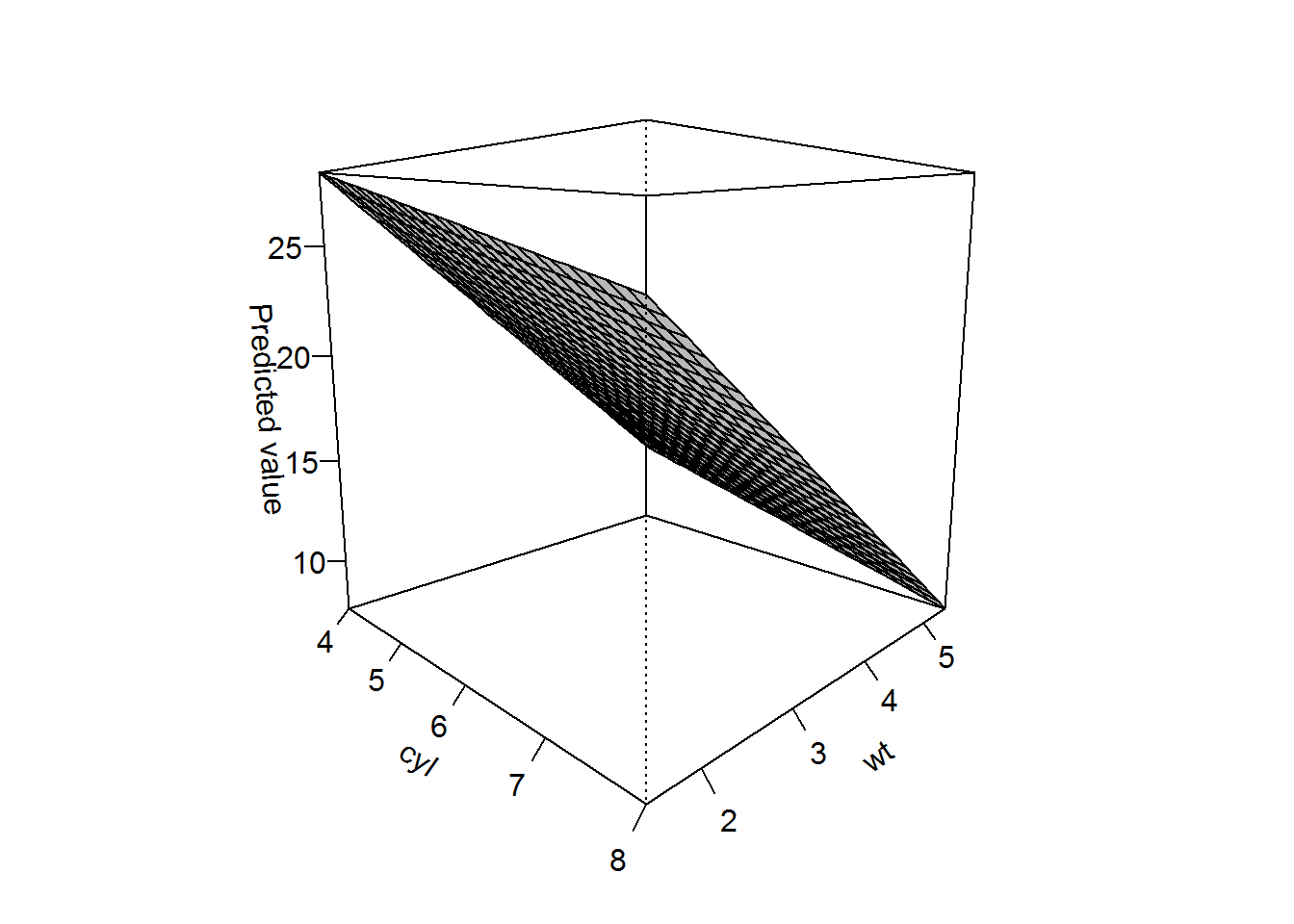This three-dimensional representation can be easily rotated using the `theta` and `phi` arguments. If multiple values are specified for each, facetting is automatically provided:

``persp(x, "cyl", "wt", theta = c(0, 90))``